## Pratt Certificate

A primality certificate based on Fermat's Little Theorem Converse. Although the general idea had been well-established for some time, Pratt became the first to prove that the certificate tree was of polynomial size and could also be verified in polynomial time. He was also the first to observe that the tree implies that Primes are in the complexity class NP.

To generate a Pratt certificate, assume thatis a Positive Integer and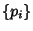is the set of Prime Factors of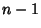. Suppose there exists an Integer(called a Witness'') such thatbut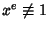(mod) wheneveris one of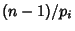. Then Fermat's Little Theorem Converse states thatis Prime (Wagon 1991, pp. 278-279).

By applying Fermat's Little Theorem Converse toand recursively to each purported factor of, a certificate for a given Prime Number can be generated. Stated another way, the Pratt certificate gives a proof that a numberis a Primitive Root of the multiplicative Group (mod) which, along with the fact thathas order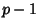, proves thatis a Prime.The figure above gives a certificate for the primality of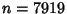. The numbers to the right of the dashes are Witnesses to the numbers to left. The setforis given by. Since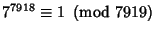but,,(mod 7919), 7 is a Witness for 7919. The Prime divisors ofare 2, 37, and 107. 2 is a so-called self-Witness'' (i.e., it is recognized as a Prime without further ado), and the remainder of the witnesses are shown as a nested tree. Together, they certify that 7919 is indeed Prime. Because it requires the Factorization of, the Method of Pratt certificates is best applied to small numbers (or those numbersknown to have easily factorable).

A Pratt certificate is quicker to generate for small numbers than are other types of primality certificates. The Mathematica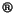(Wolfram Research, Champaign, IL) task ProvablePrime[n] therefore generates an Atkin-Goldwasser-Kilian-Morain Certificate only for numbers above a certain limit (by default), and a Pratt certificate for smaller numbers.

References

Pratt, V. Every Prime Has a Succinct Certificate.'' SIAM J. Comput. 4, 214-220, 1975.

Wagon, S. Mathematica in Action. New York: W. H. Freeman, pp. 278-285, 1991.

Wilf, H. §4.10 in Algorithms and Complexity. Englewood Cliffs, NJ: Prentice-Hall, 1986.